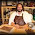## Friday, February 6, 2015

### Determining a clocks pendulum length

I am well aware that when I explain to people about the amount of math and formula work for determining work that needs done on a clock movement or to determine the proper replacement parts for a clock movement, many people do not believe me or feel I "go in to deep" to determine the answer.  For those neigh sayers I provide the following from one of my reference books for determining the length of a clocks pendulum.  Clock work, when done correctly, is very intense and I am dedicated to completing the task correctly.

INTRODUCTION:
The most common method to adjust the correct pendulum length found in clock literature involves counting teeth and pinions to calculate how many revolutions per hour the escape wheel must make, followed by determining how many beats per hour the clock was designed to make and therefore how long the pendulum should be. A worked example of this is below. A table showing numerous gear combinations is on page 59 of Practical Clock Repairing (De Carle); it shows the correct pendulum lengths for those gear combinations. Another table showing beats per minute and pendulum length is on page 79 of the same. These table make calculations much easier. However, not all gear combinations are listed, so I have chosen a common clock, the gear combination of which is not listed, for a worked example, (method #1), that assumes no available information.

Note, however, that the pendulum length is theoretical. It measures the distance from the axis of rotation to the center of gravity of the pendulum, not to the bottom of the pendulum. Further, if the clock uses a suspension spring, the thicker the spring, the faster the clock will run, so a longer pendulum would be needed.

So how do we calculate the pendulum length without counting teeth, (a tedious, error-prone procedure), nor trying to determine how much longer the practical length is than the theoretical length ?

Method #1: Tooth count method
e.g. Seth Thomas #89 movt. (note that the actual pendulum length would be longer than the theoretical length because of the thick suspension spring used on this type of clock). Here we calculate the theoretical length.

Gear Teeth Pinion Ratio R.p.h. R.p.h.
Centershaft 26 10 1 1 1
2nd Wheel 60 8 26÷60=0.433 0.6x1 =0.6
3rd Wheel 42 8 60÷8 =7.5 7.5x0.433 =3.25
4th Wheel 45 7 42÷7 =6 6x3.25 =19.5
Escape Wheel 39 7 45÷7 =6.4 6.4x19.5 =125.36

39 x 125.36 = 4889 escape teeth per hour
4889 x 2 = 9778 beats per hour
9778 ÷ 60 = 163 beats per minute

Using the formula: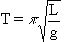(Practical Clock Repairing, De Carle, p. 77)

where T = seconds per beat
p = a constant 3.141592654
L = pendulum length in metres
g = gravity 9.81 m/s2

163 b.p.m. = 2.72 beats per second = 0.368 seconds per beat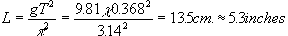Method #2: Ratios using practical length.
First example:
A clock comes in for repair without a pendulum and no clues as to its length. Make up a pendulum as long as the case will allow, or up to 39.15 inches long (a one second pendulum). Set the time, and take a reading after 24 hours. Record the error and the length of the pendulum (for example, from the middle of the suspension spring to the middle of the pendulum bob).
e.g. 39 inches long
lost one hour, 23 minutes in 24 hours.

1:23 = (1x60x60) + (23x60) = 4980 secs.
so actual reading = (24x60x60) - 4980 = 81420 secs.
desired reading = (24x60x60) = 86400 secs. (i.e. 24 hrs.)
then divide by 24 hours to convert to ratios, using a common denominator:
81420 ÷ 86400 = 0.942361
and 86400 ÷ 86400 = 1

Rearranging the formula:Square both sides: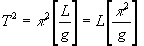Eliminate constants: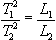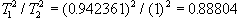The pendulum length therefore needs to be 89 % of its actual length:

39 x 0.88804 = 34.6 inches

I would then screw the pendulum bob up as high as it will go, and shorten the pendulum to 35 inches. This is because it is better to have the pendulum a little too long than too short. It can be shortened a little more if it still runs too slow.

Second example:
A clock comes in for repair with a replacement pendulum. A test run reveals that the clock gains 57 minutes in 11 hrs. 13 mins. How much longer does the pendulum need to be?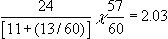2.03 hours fast per day.

The pendulum length as tested was 19 cm.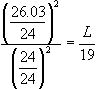L=22.35 cm.

The pendulum needs to be lengthened to 22.35 cm.

Method #3: Using the Time Trax machine.
Using the same example. While the above clock is on a test run, Time Trax readings are taken. The machine is set to take readings of 20 beats at a time, which yields fairly rapid yet consistent (low error) readings. Since the readings follow a variance pattern, readings are taken until two are the same:

 8669 8641 8652 8650 8637 8654 8667 8643 8652 8653 8631 8647

x = (8650+8637+8654+8667+8643+8652) ÷ 6 = 8650.5
The average reading eliminates these variances.

Ratios again:

(24 + 2.03)/24 = 8650.5 ÷ X

X = (8650.5 x 24)÷26.03 = 7975.05

The pendulum is lengthened until the Time Trax machine gives an average reading of 7975 beats per hour. However, the variance problem will make adjustment with accuracy difficult. In addition, the machine measures momentary performance, and performance will vary over a week if the clock is an 8 day spring-driven clock, so some additional adjustment will be necessary during a test run.

Method #4: a non - mathematical approach.
Make up a pendulum as long as the case will allow, or 39 inches, and screw the bob up as high as possible. If, on a test run, the clock runs slow, shorten the pendulum at the top by the same length as the length of thread remaining under the bob. Repeat this until the clock runs a little fast and then adjust the bob down the thread until it keeps time.

The only disadvantage with this method is that it may require for the pendulum to be shortened as many as six times or more, rather than once or twice using one or more calculation methods.

EXAMPLE 1:
Consider, in method #2, a clock that came in for repair without a pendulum. When we put it on a test run with a 39 inch pendulum, it lost 1 hr 23 mins in 24 hours. Looking at the table below:

dT is the number of minutes gained (+) or lost (-) in 24 hours.
dL is the percentage by which the pendulum needs to be lengthened by or shortened to.
The last column shows the factor by which the pendulum length reading is to be multiplied to obtain the desired length.

So dT = -83 mins in 24 hours. From the table, (where dT=-85), the pendulum is to be shortened to 89 percent of its original length, so we multiply the length by the factor of 0.89: 39 x 0.89 = 34.7 inches. Shorten the pendulum as outlined in method #2. Be conservative!

EXAMPLE #2:
Consider the second example in method #2. The pendulum is 19 cm. long and gains 2.03 hours per day ( or 121.8 minutes per day), therefore dT = + 121.8.
From the table, (where dT =+120), the pendulum needs to be lengthened by 17.36 %, so we multiply the length by the factor of 1.17:

19 x 1.17 = 22.2 cm

The pendulum needs to be lengthened to 22 cm. Note from the smaller values on dT in the tables that calculations like these are not practical for small values of dT.1.1.